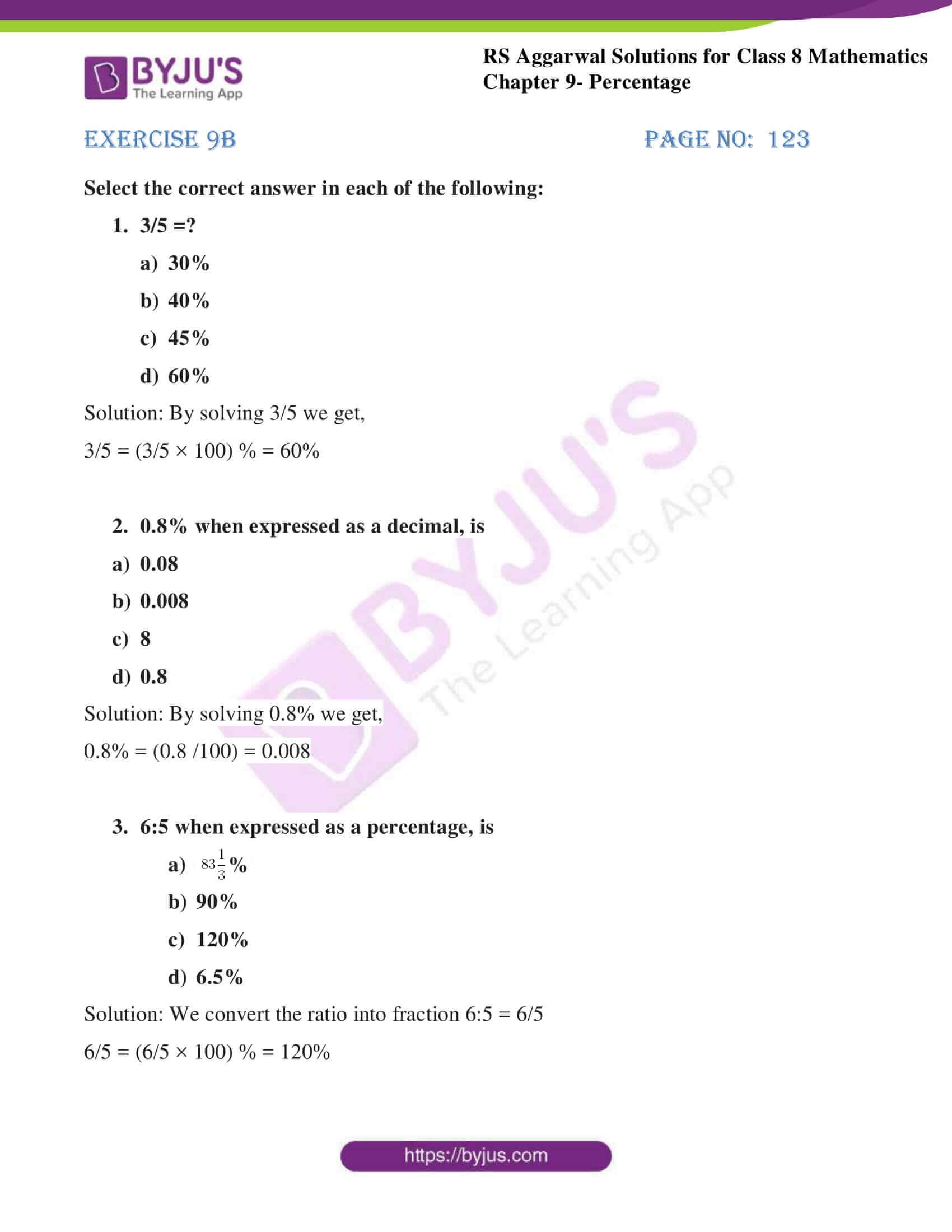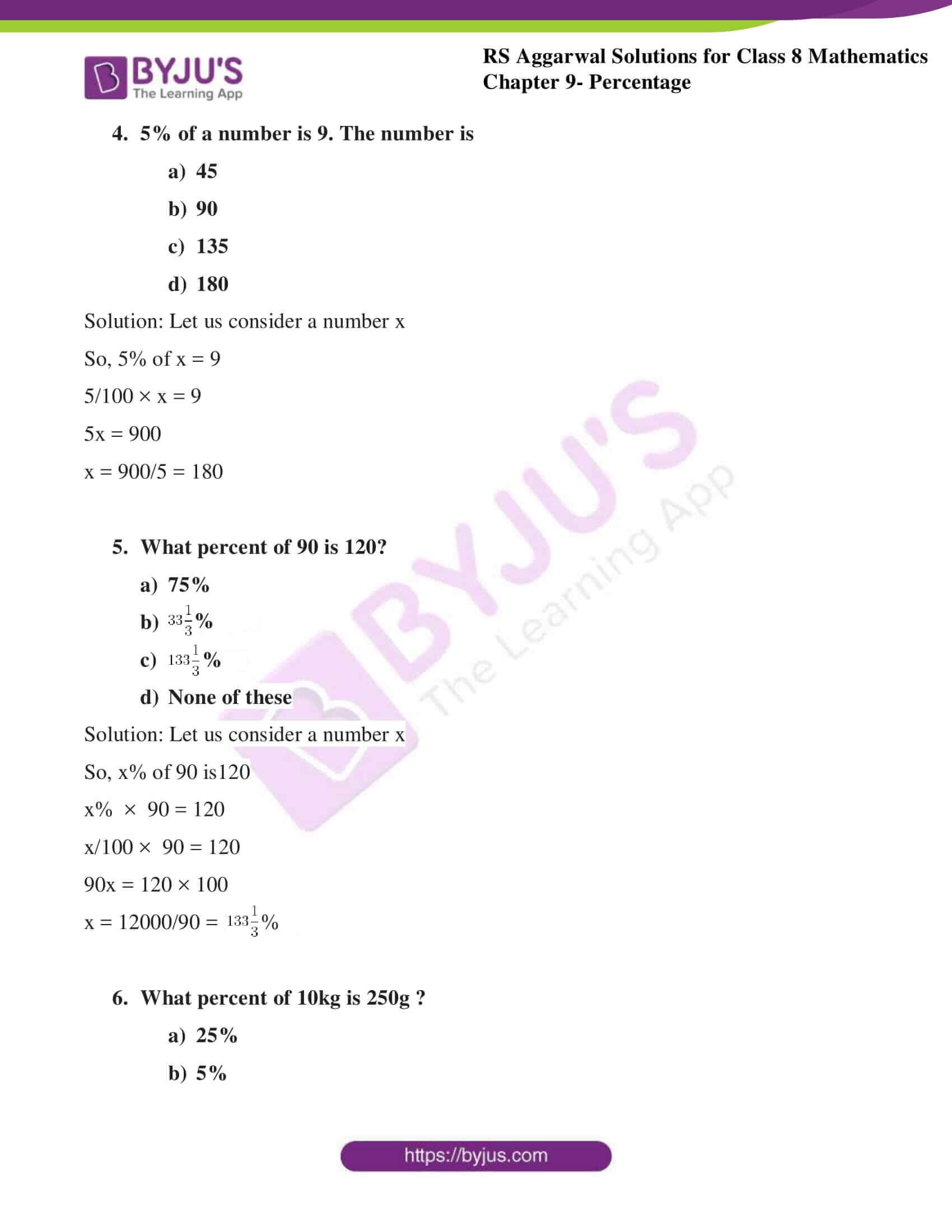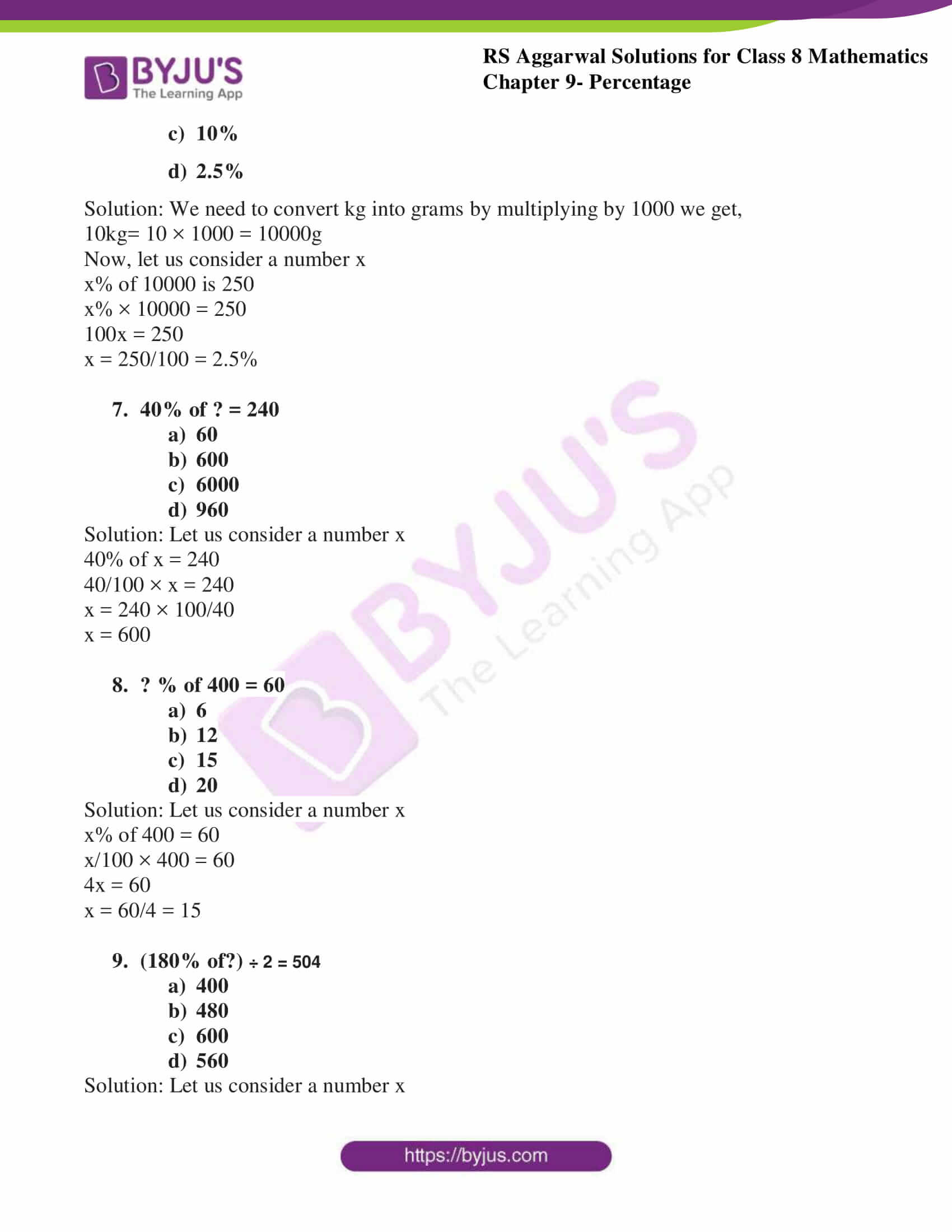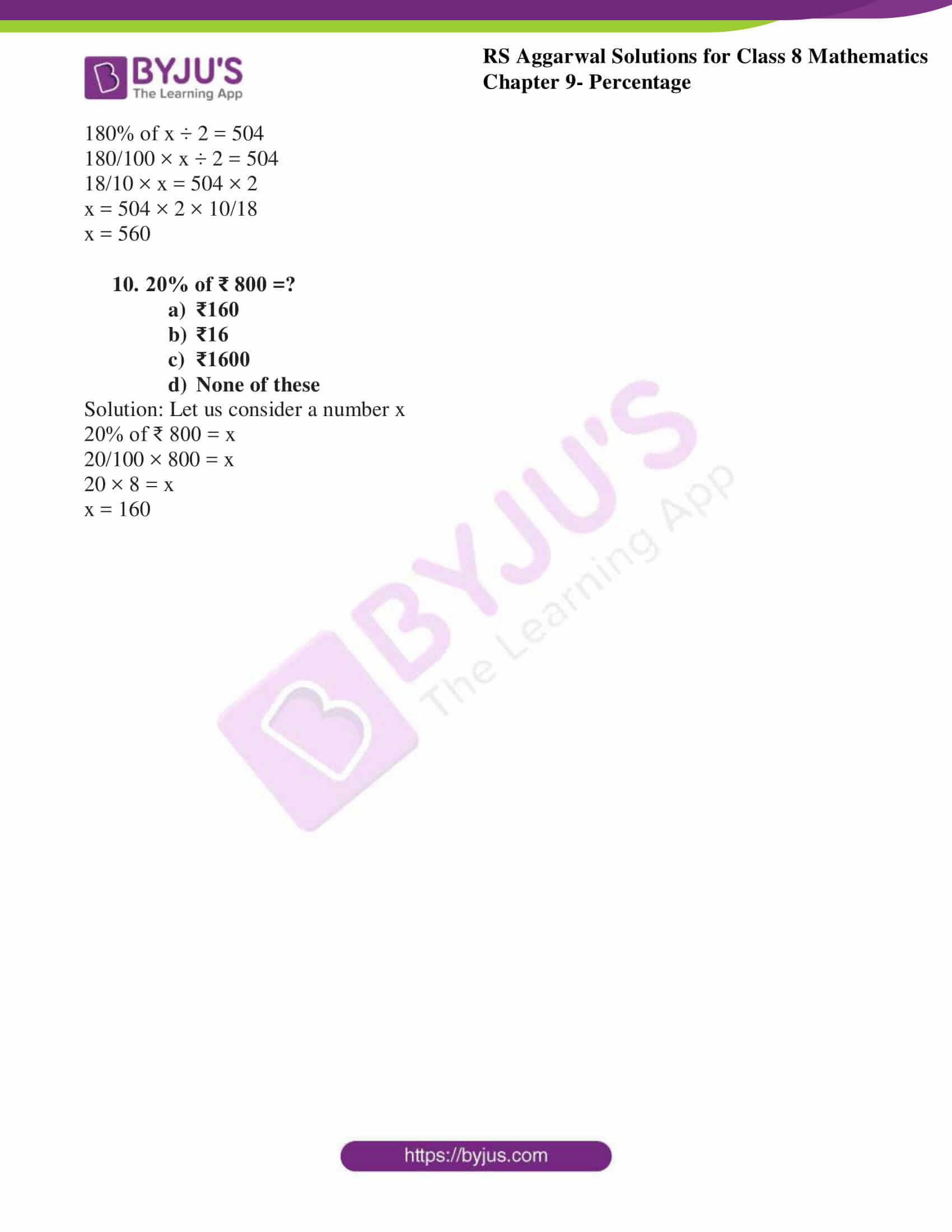# RS Aggarwal Solutions for Class 8 Maths Chapter 9 - Percentage Exercise 9B

Students can refer and download RS Aggarwal Solutions for Class 8 Maths Chapter 9- Exercise 9B, Percentage from the links provided below. Our experts have solved the RS Aggarwal Solutions to ensure that the students are thorough with their basic concepts by practicing the solutions. In Exercise 9B of RS Aggarwal Class 8 Maths, we shall see more problems based on percentage.

## Download PDF of RS Aggarwal Solutions for Class 8 Maths Chapter 9- Percentage Exercise 9B### Access Answers to RS Aggarwal Solutions for Class 8 Maths Chapter 9- Percentage Exercise 9B

Select the correct answer in each of the following:

1. 3/5 =?

1. 30%
2. 40%
3. 45%
4. 60%

Solution:

By solving 3/5 we get,

3/5 = (3/5 × 100) % = 60%

2. 0.8% when expressed as a decimal, is

1. 0.08
2. 0.008
3. 8
4. 0.8

Solution:

By solving 0.8% we get,

0.8% = (0.8 /100) = 0.008

3. 6:5 when expressed as a percentage, is

1. $83\frac{1}{3}$%
2. 90%
3. 120%
4. 6.5%

Solution:

We convert the ratio into fraction 6:5 = 6/5

6/5 = (6/5 × 100) % = 120%

4. 5% of a number is 9. The number is

1. 45
2. 90
3. 135
4. 180

Solution:

Let us consider a number x

So, 5% of x = 95/100 × x = 9

5x = 900

x = 900/5 = 180

5. What percent of 90 is 120?

1. 75%
2. $33\frac{1}{3}$%
3. $133\frac{1}{3}$%
4. None of these

Solution:

Let us consider a number x

So, x% of 90 is120

x% × 90 = 120

x/100 × 90 = 120

90x = 120 × 100

x = 12000/90 = $133\frac{1}{3}$%

6. What percent of 10kg is 250g ?

1. 25%
2. 5%
3. 10%
4. 2.5%

Solution:

We need to convert kg into grams by multiplying by 1000 we get,

10kg= 10 × 1000 = 10000g

Now, let us consider a number x

x% of 10000 is 250

x% × 10000 = 250

100x = 250

x = 250/100 = 2.5%

7. 40% of ? = 240

1. 60
2. 600
3. 6000
4. 960

Solution:

Let us consider a number x

40% of x = 240

40/100 × x = 240

x = 240 × 100/40

x = 600

8. ? % of 400 = 60

1. 6
2. 12
3. 15
4. 20

Solution:

Let us consider a number x

x% of 400 = 60x/100 × 400 = 60

4x = 60

x = 60/4 = 15

9. (180% of?) ÷ 2 = 504

1. 400
2. 480
3. 600
4. 560

Solution:

Let us consider a number x

180% of x ÷ 2 = 504

180/100 × x ÷ 2 = 504

18/10 × x = 504 × 2

x = 504 × 2 × 10/18

x = 560

10. 20% of ₹ 800 =?

1. ₹160
2. ₹16
3. ₹1600
4. None of these

Solution:

Let us consider a number x

20% of ₹ 800 = x

20/100 × 800 = x

20 × 8 = x

x = 160

### Access other Exercise of RS Aggarwal Solutions for Class 8 Maths Chapter 9- Percentage

Exercise 9A Solutions 23 Questions

## RS Aggarwal Solutions for Class 8 Maths Chapter 9- Percentage Exercise 9B

Exercise 9B of RS Aggarwal Class 8, Percentage. This exercise mainly deals with Objective questions related to the entire Chapter.

The RS Aggarwal Solutions with step by step answering ensures that students to get to know the best approach to the individual questions. By referring to these solutions, students develop problem-solving abilities and can tackle any type of questions easily. Students are suggested to practice the problems on a regular basis which will help them excel in their exams and increase their overall percentage. Practicing as many times as possible helps in time management skills and also boosts the confidence level to achieve high marks.